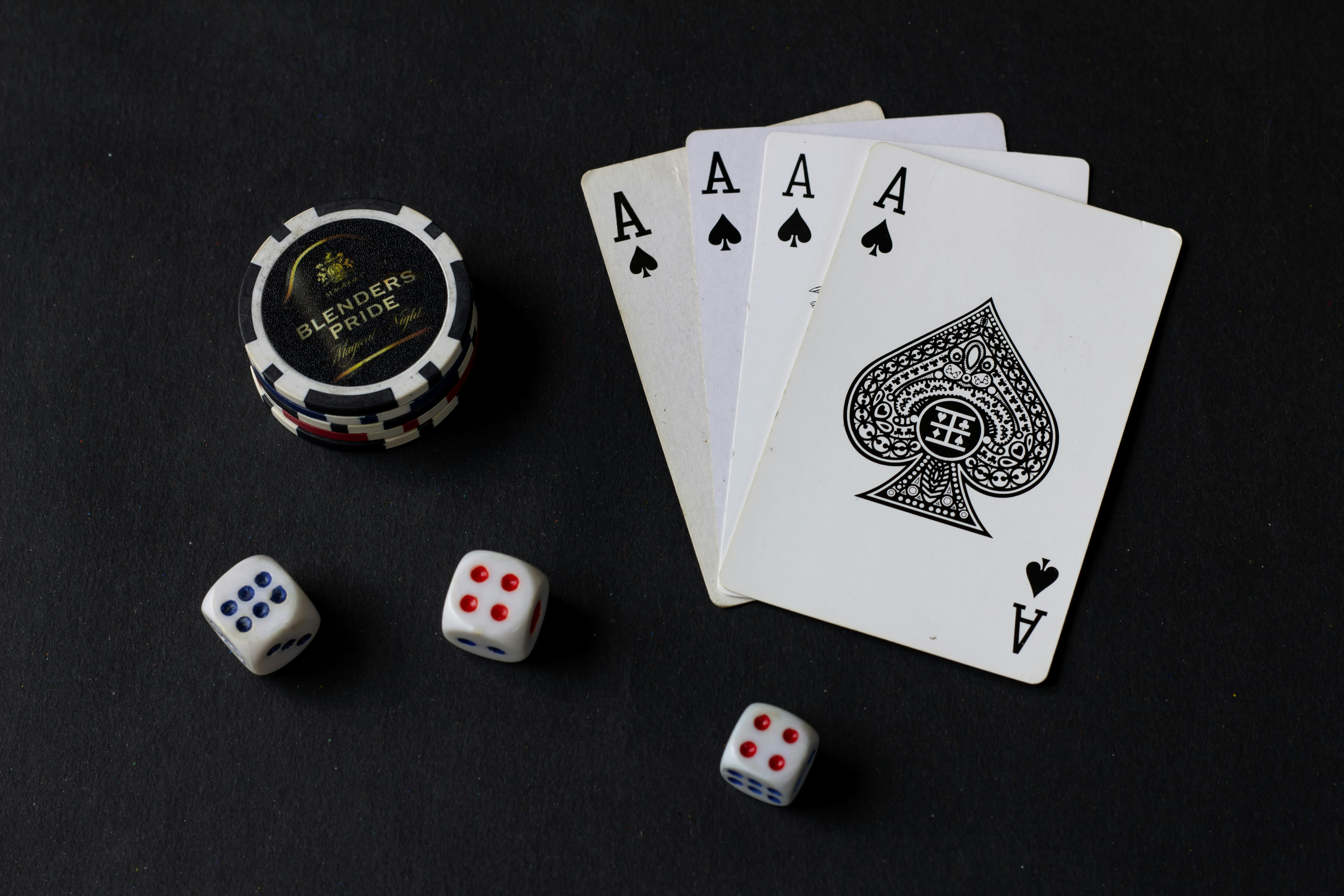# Simulations In Probability

## Destined Probable Chances In Randomized Action

By Yoel DavidsonPublished 3 months ago 1 min read
LikePhoto by aceofnet on Unsplash

Using Simulations In Probability

I use simulations to study phenomenas too complex to analyze by other means. Estimations, not determine, use in probable simulations.

Random-digit tables are lists of digits selected at random, often by computer or calculator. To simulate a coin toss, pick a number at random to start and then read across the table, letting an even digit represent heads and an odd digit represent tails. Continue this process for 100 digits.

The simulated probability of heads is the ratio of the number of even digits found (heads) to 100. If even numbers represent heads, for example, the simulated probability of tossing a head is P(H)= 44/100. When the number of random digits chosen is much greater, then the simulated probability approaches the theoretical probability.

To simulate whether an aspiring pet parent will adopt two Labrador Retriever puppies or two Chihuahuas, one may employ a random-digit table with an even (pair of) digits representing two Labrador Retriever puppies and an odd (pair of) digits representing two Chihuahuas, because the adoption is for two puppies. If we examine 100 pairs, then the simulated probability of two puppies will be the number of pairs of even digits divided by 100, the total number of pairs considered.

performance poetrysocial commentarynature poetryinspirationalfact or fictionart
Like

### Yoel Davidson

How does it work?

There are no comments for this story

Be the first to respond and start the conversation.